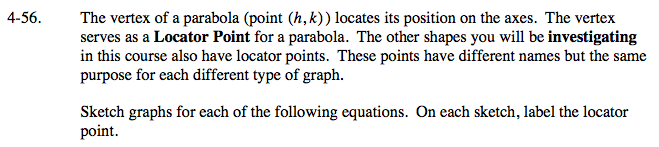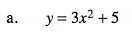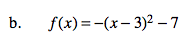### Home > A2C > Chapter 4 > Lesson 4.1.4 > Problem4-56

4-56.
1. The vertex of a parabola (point (h, k)) locates its position on the axes. The vertex serves as a Locator Point for a parabola. The other shapes you will be investigating in this course also have locator points. These points have different names but the same purpose for each different type of graph.

Sketch graphs for each of the following equations. On each sketch, label the locator point. 4-56 HW eTool (Desmos). Homework Help ✎

1. y = 3x2 + 5

2. f (x) = −(x − 3)2 − 7(0, 5)(3, −7)

Use the eTool below to view the graphs of the equations.
Click the link at right for the full version of the eTool: A2C 4-56 HW eTool